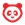# Community

cancel
Showing results for
Show  only  | Search instead for
Did you mean:

# New Quizzes Formula Question / Pick from set

There needs to be a way to set up a formula question so that a variable can pick from a set, rather than simply being assigned a random value in a range.  For example, if I wrote a question which says "what is 5 plus `x`?", I only have the option of giving a min and max value for x. If min=5 and max=10, then Canvas randomly picks values from 5-10 as expected.

While I like that functionality on some questions, what I would like to see is where I could optionally tell Canvas a set of values to randomly pick from.  For example, in my question above, have X be set to a value that is randomly picked from this set: {1, 2, 4, 8, 16}Community Team
Status changed to: Open for ConversationCommunity Member

Or, at the very least be able to exclude values from the range.  When a problem involves division of positive and negative values, I have to do 4 versions of the problem for +/+, +/-, -/=, and -/-.  Otherwise I run the risk of dividing by 0. (I know I could allow for 0 in the numerator, but where's the challenge in 0/x?).

Related issues:

Showing addition and subtraction based on the sign of the random value:  In the formula x + `b`, it would look fine as long as b is positive.  However, if b were -3, it would show up as x + -3.  Is there a way for the formula to recognize that b is negative and either change the + to -, x - 3, or put b in parentheses, x + (-3)?

Coefficients of 1 and -1:  When using variable values as coefficients, could the formula recognize coefficients of 1 or -1 and not show them?  We don't generally write the 1's.  By the same token, exponent of 1 and index of 2.

These threads tie in with this one:

Variables used by multiple questions: https://community.canvaslms.com/t5/Idea-Conversations/Grouped-Formula-Questions-Shared-Set-of-Define...